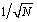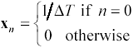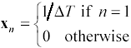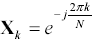# Checking the Output of Your FFT Routine

Before using an unfamiliar FFT routine, you should always check the transform of a simple impulse at time 0 and also the transform of the same impulse delayed by one sample. Table 4.3 shows the results you should obtain. These checks will establish that you have used the correct scaling factor. It is common to find FFT routines that scale the forward transformation by 1/ N ,, or 1, a simple problem to correct.

The checks also establish the direction of frequency rotation in the complex plane. Some FFT routines pervert the kernel of the transformation by using a plus j instead of a minus j in the exponent. This change inverts the frequency axis in the forward transform and reverses the time axis in the inverse direction, as compared to the transformation described in [4.3].

If your transform uses a plus- j kernel, you can fix it by forming the complex conjugate of the frequency domain result after running the perverted transform. The conjugation gives you the answer you should have gotten with a proper transform. In the reverse direction you must remember to conjugate each correct frequency-domain vector before submitting it to the perverted inverse transform for calculation of time-domain results. Alternately, you could use the perverted reverse transform for the forward transform, and vice versa, with attention paid to the scaling factor of N in both directions.

Table 4.3. FFT Test Cases

Object

Time-domain

Frequency-domain

impulseX k = 1

shifted impulsePOINT TO REMEMBER

• Before using an unfamiliar FFT routine, check the transform of a simple impulse at time 0 and also the transform of the same impulse delayed by one sample.

For further study see: www.sigcon.comHigh-Speed Signal Propagation[c] Advanced Black Magic
ISBN: 013084408X
EAN: N/A
Year: 2005
Pages: 163